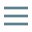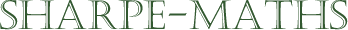Previous topic:
Current topic:
Algebraic Fractions
Next topic:
Simple linear equations

### Algebraic Fractions

The important thing to understand about these, is that the techniques you have learnt to deal with numeric fractions and all going to work here as well. In some ways, you could argue that algebraic fractions are easier than numeric ones.

Suppose we are adding the fractions $\frac{3}{8}+\frac{1}{6}$, we have to find the LCM of $8$ and $6$ which is $24$.

However this problem $\frac{1}{x}+\frac{2}{y}$ is much easier to get going with as the LCM of $x$ and $y$ is simply $xy$. No calculation required at all.

If you are still not confident with numerical fractions, please go back and revise that topic before moving on with this one.

In addition to understanding how numerical fractions work, it is also essential to have quadratic factorisation sorted out in your head as well. The first thing you should ask, when simplifying or otherwise operating on algebraic fractions, is Can I factorise anything?. If the answer is Yes then that is what you should do first. If you follow this principle, you will be fine.

### Mucking about with minus signs

One part of working with fractions comes from a confusion with the possible positions of minus signs, which I will try to deal with here. Look at the following fractions:

$-\frac{a}{b}=\frac{-a}{b}=\frac{a}{-b}$

These all mean the same thing. There is no difference in mathematical meaning between them other than the obvious notation difference. Below, I try to explain in algebraic notation, why this is so.

$\frac{-a}{b} = \frac{-1 \times a}{b} = \frac{-1 \times a \times -1}{b \times -1} = \frac{a}{-b}$

The second fraction is the same as the first except that $-a$ is written as $-1 \times a$. In the third fraction along, I have multiplied the numerator and denominator by $-1$, so that is an equivalent fraction. The final fraction is found by simplifying top and bottom.

In the same way, we can examine how to get from $-\frac{a}{b}$ to $\frac{-a}{b}$, as follows:

$-\frac{a}{b} = \frac{-1}{1} \times \frac{a}{b} = \frac{-a}{b}$